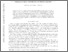# Quadratic principal indecomposable modules and strongly real elements of finite groups

Gow, Roderick and Murray, John (2019) Quadratic principal indecomposable modules and strongly real elements of finite groups. Proceedings of the American Mathematical Society, 147. pp. 2783-2796. ISSN 1088-6826Previewmore...Add this article to your Mendeley library

## Abstract

Let P be a principal indecomposable module of a finite group G in characteristic 2 and let φ be the Brauer character of the corresponding simple G-module. We show that P affords a non-degenerate G-invariant quadratic form if and only if there are involutions s,t∈G such that st has odd order and φ(st)/2 is not an algebraic integer. We then show that the number of isomorphism classes of quadratic principal indecomposable G-modules is equal to the number of strongly real conjugacy classes of odd order elements of G.

Item Type: Article This is the preprint version of the published article, which can be cited as: arXiv:1803.03182. T Quadratic principal; indecomposable; modules; strongly real elements; finite Groups; Faculty of Science and Engineering > Mathematics and Statistics 13520 https://doi.org/10.1090/proc/14441 Dr. John Murray 11 Nov 2020 15:45 Proceedings of the American Mathematical Society American Mathematical Society YesItem control page